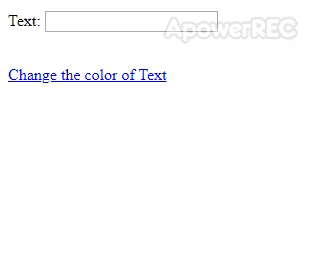# How to use JavaScript variables in jQuery selectors ?

In this article, we will discuss how to use JavaScript variables in jQuery selectors. In the following examples, it can be seen that we have used the values stored in JavaScript Variables are used inside the jQuery Selectors.

Example 1: The concatenation technique can be applied in order to use the values stored in JavaScript variables. In the following example, whenever the button is clicked, the content present inside the <span> element is appended to the <p> element. Then we will use the ready() method that helps to load the whole page then execute the rest code.

 ` ` ` `  `<``html``> ` ` `  `<``head``> ` `    ``<``title``>JavaScript variables in jQuery slectors ` ` `  `    ``<``script` `src``=``"https://code.jquery.com/jquery-3.4.1.js"` `            ``integrity``= ` `"sha256-WpOohJOqMqqyKL9FccASB9O0KwACQJpFTUBLTYOVvVU="`  `            ``crossorigin``=``"anonymous"``> ` `    `` ` ` ` ` `  `<``body``> ` `    ``<``div``> ` `        ``<``button` `id``=``"button"` `                ``style``=``"color: green;"``> ` `            ``Click Me ` `        `` ` ` `  `        ``<``p` `id``=``"firstpara"``> ` `            ``<``span` `id``=``"span"`  `                  ``style``=``"color: green;"``> ` `                ``A Computer Science Portal fo Geeks<``br``>  ` `            `` ` `        `` ` `    `` ` `    ``<``script``> ` `        ``\$(document).ready(function() { ` `            ``\$("#button").click(function() { ` `                ``var paraId = "firstpara"; ` `                ``var spanId = "span"; ` `                ``\$("#" + paraId).append(\$("#" + spanId).html()); ` `            ``}); ` `        ``}) ` `    `` ` ` ` ` `  ` `

Output:Example 2: The following example changes the color of the text when the link is pressed. In this example, javascript:void(0); is used inside <a> element. Then we will use the ready() method helps to load the whole page then execute the rest code.

 ` ` `<``html``> ` ` `  `<``head``> ` `    ``<``title``>JavaScript variables in jQuery slectors ` ` `  `    ``<``script` `src``=``"https://code.jquery.com/jquery-3.4.1.js"` `            ``integrity``= ` `"sha256-WpOohJOqMqqyKL9FccASB9O0KwACQJpFTUBLTYOVvVU="`  `            ``crossorigin``=``"anonymous"``> ` `    `` ` ` ` ` `  `<``body``> ` `    ``<``form``> ` `        ``<``p``>  ` `          ``Text: ` `          ``<``input` `type``=``"text"``>  ` `        `` ` `        ``<``br``> ` ` `  `        ``<``a` `href``=``"javascript:void(0);"``> ` `          ``Change the color of Text  ` `        `` ` `    `` ` `    ``<``script``> ` `        ``\$(document).ready(function() { ` ` `  `            ``\$("a").click(function() { ` `                ``var type = \$("input").attr("type"); ` `                ``var attribute = "color"; ` `                ``var color = "green"; ` ` `  `                ``\$("input[type=" + type + "]") ` `                ``.css(attribute, color); ` `            ``}); ` `        ``}) ` `    `` ` ` ` ` `  ` `

Output:My Personal Notes arrow_drop_upCheck out this Author's contributed articles.

If you like GeeksforGeeks and would like to contribute, you can also write an article using contribute.geeksforgeeks.org or mail your article to contribute@geeksforgeeks.org. See your article appearing on the GeeksforGeeks main page and help other Geeks.

Please Improve this article if you find anything incorrect by clicking on the "Improve Article" button below.

1

Please write to us at contribute@geeksforgeeks.org to report any issue with the above content.